# How to Tackle Every Single GMAT Problem (Seriously!) - Part 1

by , Apr 18, 2015Wouldnt it be nice to have one common thread among all GMAT problems youll ever do, something you do no matter what kind of problem or content area is being tested?

Okay, obviously, there are many things youre going to need to learn and practice in order to get a great GMAT scorethere isnt literally just one thing to learn. But it turns out that there is one set of principles tying together everything we need to do on the GMAT.

Here it is: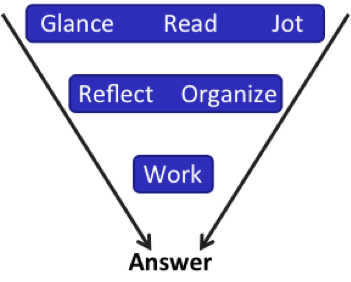You can use this on Problem Solving (PS) and Data Sufficiency (DS) problems. Itll work on Sentence Correction (SC), Reading Comprehension (RC), and Critical Reasoning (CR). It even works for Integrated Reasoning (IR) and the essay! And Im going to show you how.

Try this PS problem from the GMATPrep free exams.

*The perimeter of a certain right isosceles triangle is [pmath]16+16sqrt{2}[/pmath]. What is the length of the hypotenuse of the triangle?

(A) 8

(B) 16

(C) [pmath]4sqrt{2}[/pmath]

(D) [pmath]8sqrt{2}[/pmath]

(E) [pmath]16sqrt{2}[/pmath]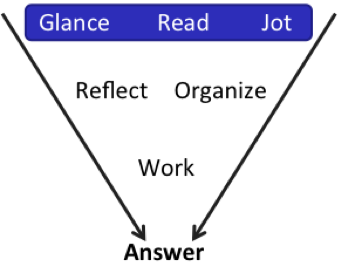Glance is literally what it means. Take about 1-2 seconds to just glance and see what you have.

• Is it PS or DS?

Right now, youre rolling your eyes at me. A fellow teacher of mine did an experiment last year. She put a math question up in class for a few seconds, then pulled it off and asked the class whether the problem was PS or DS. Fewer than half of the students were able to say! People are so anxious to start reading that they dont even take in the whole screen to see what theyve got.

So your first task is to notice, consciously, whether you have PS or DS. This one is PS. Next:

• Stories / lots of words? Numbers / formulas? Graphics?

Theres a weird number in the middle there. Dont start reading yet! One more thing:

• What are the answers like?

Hmm. Two are nice numbers and three are like the weird number in the problem. Youre not going to do anything with that knowledge yet, but do remember it.

Okay, finally, you can go ahead and Read this thing. As you read, Jot down the major information.

Note: jot means just that: write down whats there, but do not start solving or manipulating anything yet. Just get everything on your paper.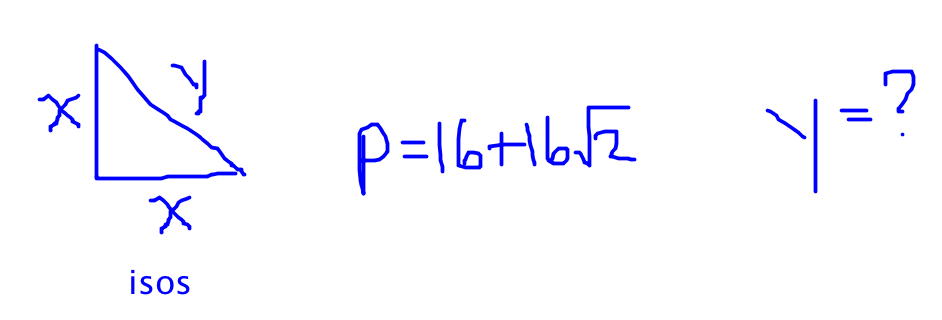They told me its a right triangle and its isosceles, so I drew a little picture. After a second of thought, I added the labels to remind myself that its isosceles. Then they gave me that equation and they asked me for the hypotenuse, which Id labeled y.

I have not yet thought about how Im going to solve this thing. Im not at that stage yet! This is one of the big differences between someone who does really well on quant and someone who knows a lot of stuff but is struggling to put it together: you actually want to take your time to figure out your options before you decide how to solve.

Now, its time to solve, right?

Not so fast!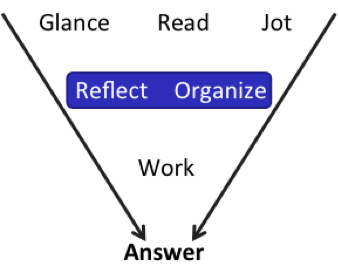Now, I reflect on what Ive got and figure out what path I want to take. If either of these first two steps / rows fail, then I know I shouldnt even bother to go to step 3. Instead, I should just pick my favorite letter and move on.

So what have we got here? Theres a right triangle, so I actually have a second formula: the Pythagorean Theorem. I can add that to my notes, using the variables that I chose: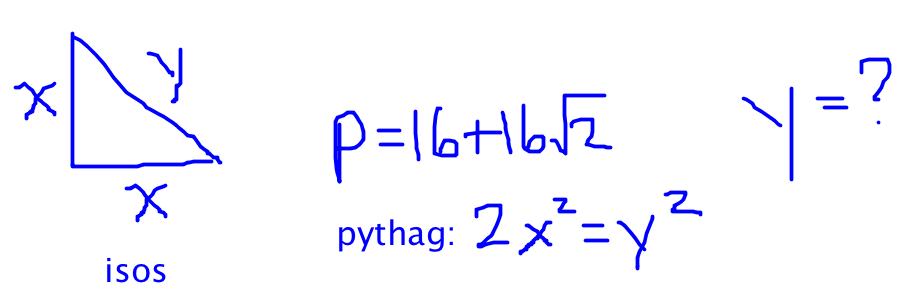The answer choices make more sense now. At least one of the sides of the triangle should include [pmath]sqrt{2}[/pmath]since the perimeter does. And two of the sides have to be the samehmm. I wonder if its as easy as saying the sides are 8, 8, and [pmath]16sqrt{2}[/pmath]? (Im spilling over into step 3, Work, here.)

Oh, no, wait, that wont work, because 8 + 8 = 16, which is smaller than [pmath]16sqrt{2}[/pmath]. The sum of the other two sides cant be smaller than the third side.

Back up: back to Reflect and Organize. That wasnt a complete waste of time, because now I know the answer is not (E). I also wouldnt guess (A) at this point, because 8 is part of the faulty calculation that I just did, so its probably not right. In fact, maybe 8 is too small in general. I could probably estimate to get rid of some of the other answers. Something to keep in mind if I cant come up with a better plan.

I could also work backwards, just trying the answers in the problem. Thatd be pretty easy for (A) and (B), but the other two remaining answers are more annoying.

Oh, back to my original idea: I first tried to split the 16 into two parts (8 and 8), but they werent big enough to be the legs. What if I split [pmath]16sqrt{2}[/pmath]into two parts? Lets do some more Work.

In this case, the two legs would be [pmath]8sqrt{2}[/pmath]and the hypotenuse would be 16. Does that fit the Pythagorean theorem?

[pmath](8sqrt{2})^2+(8sqrt{2})^2=16^2[/pmath]

[pmath](64)(2)+(64)(2)=256[/pmath]

[pmath]128+128=256[/pmath]

Bingo! This math works, so the hypotenuse equals 16.

Right now, you may be thinking, Yeah, thats okay for you; you do really well on this test. But I cant take all that time to Reflect and Organize before I start Working!

I know you feel that waybut youre wrong! Youre nervous that youll need more time to solve, because youre used to diving in and trying to solve without a clear plan and that approach, naturally, takes a lot of time. If you can actually come up with a clear plan, your solution process will be a lot fasterI promise.

What if you cant come up with a clear plan? Then your best bet is to bail on this question. Dont start writing down random numbers / formulas and seeing where they take you, using up precious time and mental energy. Get out of the problem entirely!

Lets take a look at some of the other paths that I rejected.

## Working Backwards

You can work backwards when the answer choices represent a discrete number or variable in the problem and those answers are relatively nice numbers. In this case, (A) and (B) are very nice. The other three arent quite as nice, but they may not be terrible.

We generally recommend starting with answer (B) or (D). Answer (B) is easier, so start there.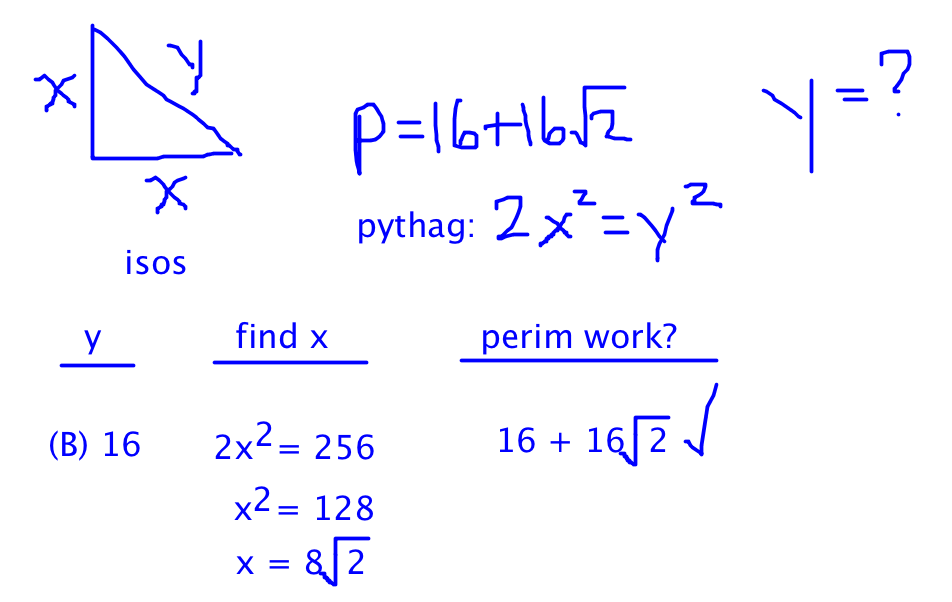Now, we got lucky, because the first one we tried worked. What if wed tried answer (D) first instead?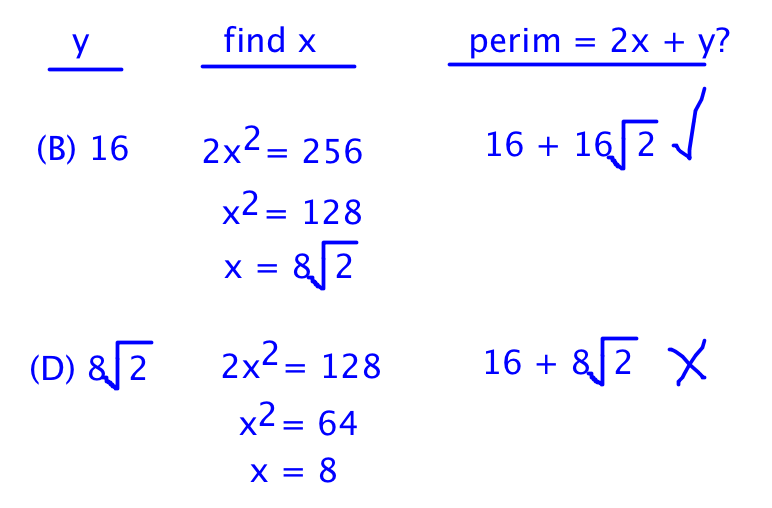The math actually isnt that horrible, after all, even though the starting number is annoying. I still like the first way I did it better, but if I hadnt thought of that, then this is a good alternative approach.

## Estimation

I also noted earlier that I might be able to eliminate some answers via estimation. If the hypotenuse is 8, for example, then the two legs have to be smaller than 8, so the total perimeter has to be less than 24. [pmath]16+16sqrt{2}[/pmath]is definitely greater than 24, so 8 cant be the hypotenuse.

What about [pmath]4sqrt{2}[/pmath]? The square root of 2 equals approximately 1.4. Call it 1.5, since were estimating. (4)(1.5) = 6, which is even smaller than 8. Answer (C) cant be correct either!

Lets try [pmath]8sqrt{2}[/pmath]. Thats about 12, so the two legs would have to be smaller, and the perimeter would have to be less than 36.

Hmm. [pmath]16+16sqrt{2}[/pmath]is about 16 + (16)(1.5) = 16 + 24 = 40. It cant be answer (D) either. Were down to 2 answer choices, just by estimating! Lets keep going.

If the hypotenuse is 16, then the perimeter has to be less than 48. That works with the 40 figure. And if the hypotenuse is [pmath]16sqrt{2}[/pmath], then the numbers could also fit.

Were down to two answers just by estimating, and if youd already figured out, as we did at the beginning, that (E) couldnt be right, then youd know the answer has to be (B)!

Keep an eye out for this series; every week, I'll be doing a new problem type using the overall GRW approach. (GRW = the first letters of each of the three lines. Maybe you can help me come up with a better name for this ...)

## Key Takeaways for Every Problem You Will Ever Do:

(1) First, you have to understand whats in front of you. Glance at the problem to pick up any clues you can about what it is, where the complexity is, and what kinds of strategies might be available to you. As you Read, Jot down the given informationbut dont try to solve yet!

(2) Next, Reflect on what youve been given and Organize your thoughts and your scrap paper. Notice how much thinking I did before I went ahead and solved the thing? I came up with three different approaches and then picked the one that seemed the best to me. And heres the best part: this valuable investment of time will help you to pick the best path when you can do so and it will help you to guess and move on when thats the best call to make.

(3) Finally, you get to do the Work to solve this thing, assuming that you actually passed the first two steps: you understand the problem and you have a plan to solve. If not, then your best move is to make an educated guess when possible (estimate, for example) or just pick your favorite letter and move on.

* GMATPrep questions courtesy of the Graduate Management Admissions Council. Usage of this question does not imply endorsement by GMAC.